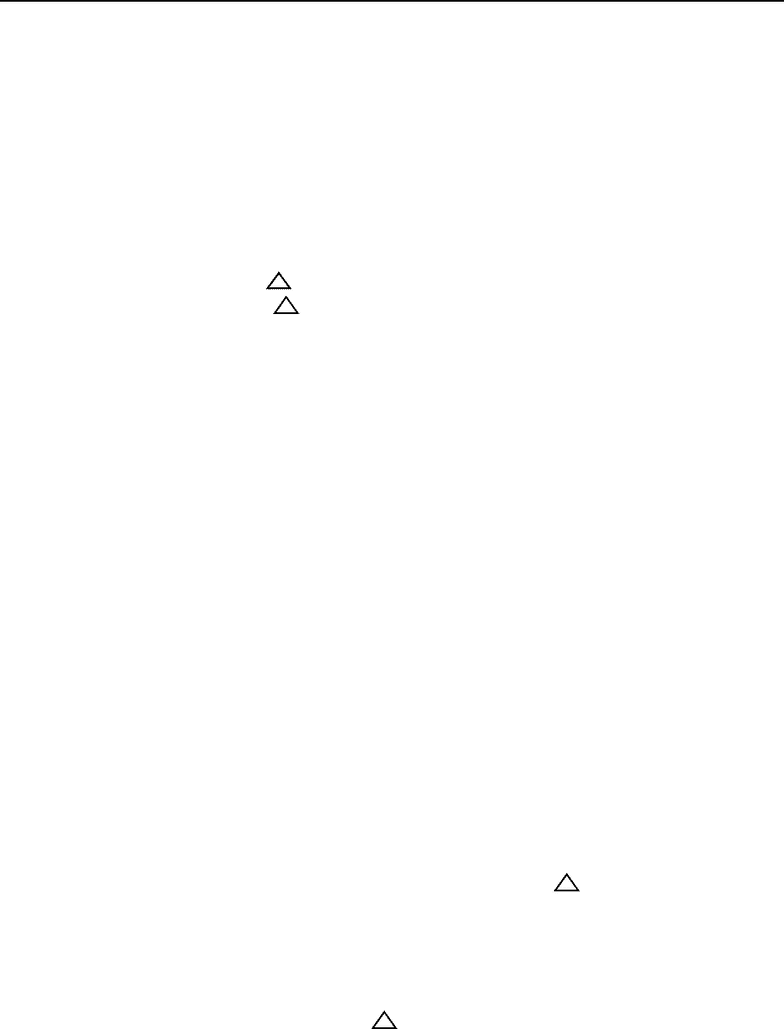# CHMA10H3 Lecture Notes - Lecture 7: Heat Capacity, Chemical Equation

91 views3 pagesCHMA10 Lecture 7: Summarizing Calorimetry and Relationships Involving Enthalpy Changes
- 6.7: Constant Pressure Calorimetry
o Commonly known as coffee cup calorimetry
o Utilized to determine the change in enthalpy of a given reaction
o Ensures that reaction takes place under constant pressure
o Common equations
qreaction = - qsolution
the insulated calorimeter prevents any heat from escaping
Therefore we can assume that the heat gained by the solution
equal the heat lost by the reaction or vice versa
qreaction = Hr
qreaction = ( Hr ) / n
to get the change in enthalpy per mole of a particular reactant,
we divide by the number of moles that actually reacted
o Coffee-Cup Calorimeter: measures enthalpy changes for chemical reactions in a
solution
Made up of two Styrofoam cups
One is inserted into the other
This setup provides insulation from the outside environment
Calorimeter is also equipped with a thermometer and stirring device
Have to ensure that the given reactions occurs in a specifically measured
quantity of solution within the calorimeter
This allows us to know the exact mass of the solution
During the reaction, any heat that is absorbed causes a temperature
change in the solution
The thermometer is needed in order to determine when this
happens and how significant the temperature change happens to
be
If we know the specific heat capacity of the solution, normally
assumed to be that of water, we can calculate the heat of the
solution using the following equation
o Represented by qsolution
o qsolution = ( msolution )( Csolution ) T
- Summarizing Calorimetry
o 1: Bomb Calorimetry
Allows you to calculate the change in internal energy of any given
reaction
Measures qv = U for a reaction
Key feature is that it occurs at constant volume
o 2: Coffee-cup Calorimetry
Unlock document

This preview shows page 1 of the document.
Unlock all 3 pages and 3 million more documents.

# Get access

\$10 USD/m
Billed \$120 USD annually
Homework Help
Class Notes
Textbook Notes